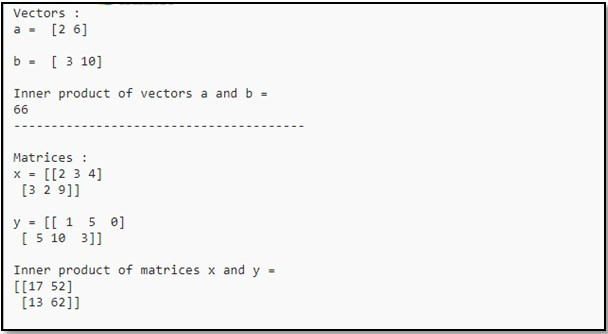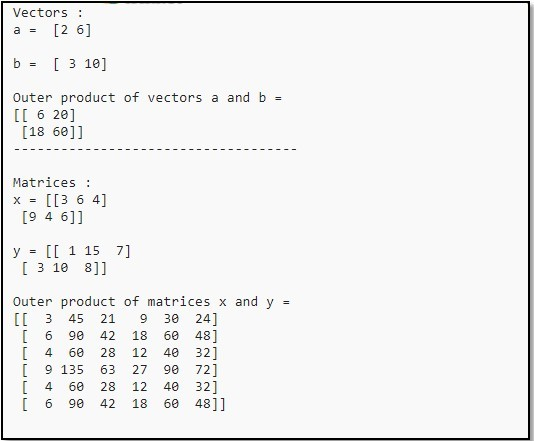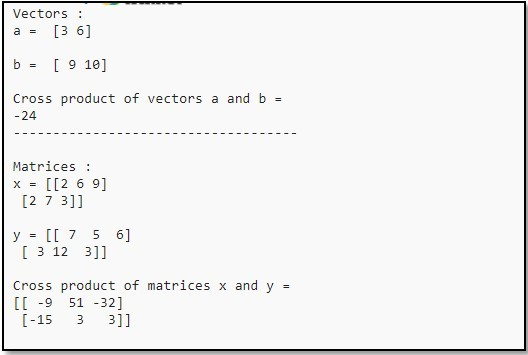GeeksforGeeks App
Open AppBrowser
Continue

# Calculate inner, outer, and cross products of matrices and vectors using NumPy

Let’s discuss how to find the inner, outer, and cross products of matrices and vectors using NumPy in Python.

Inner Product of Vectors and Matrices

To find the inner product of the vectors and matrices, we can use the inner() method of NumPy.
Syntax:

`numpy.inner(arr1, arr2)`

Code :

## Python3

 `# Python Program illustrating``# numpy.inner() method``import` `numpy as np`` ` `# Vectors``a ``=` `np.array([``2``, ``6``])``b ``=` `np.array([``3``, ``10``])``print``(``"Vectors :"``)``print``(``"a = "``, a)``print``(``"\nb = "``, b)`` ` `# Inner Product of Vectors``print``(``"\nInner product of vectors a and b ="``)``print``(np.inner(a, b))`` ` `print``(``"---------------------------------------"``)`` ` `# Matrices``x ``=` `np.array([[``2``, ``3``, ``4``], [``3``, ``2``, ``9``]])``y ``=` `np.array([[``1``, ``5``, ``0``], [``5``, ``10``, ``3``]])``print``(``"\nMatrices :"``)``print``(``"x ="``, x)``print``(``"\ny ="``, y)`` ` `# Inner product of matrices``print``(``"\nInner product of matrices x and y ="``)``print``(np.inner(x, y))`

Output :Outer Product of Vectors and Matrices

The outer product of the vectors and matrices can be found using the outer() method of NumPy.
Syntax:

`numpy.outer(a, b, out = None)`

Code :

## Python3

 `# Python Program illustrating ``# numpy.outer() method ``import` `numpy as np`` ` `# Vectors``a ``=` `np.array([``2``, ``6``])``b ``=` `np.array([``3``, ``10``])``print``(``"Vectors :"``)``print``(``"a = "``, a)``print``(``"\nb = "``, b)`` ` `# Outer product of vectors ``print``(``"\nOuter product of vectors a and b ="``)``print``(np.outer(a, b))`` ` `print``(``"------------------------------------"``)`` ` `# Matrices``x ``=` `np.array([[``3``, ``6``, ``4``], [``9``, ``4``, ``6``]])``y ``=` `np.array([[``1``, ``15``, ``7``], [``3``, ``10``, ``8``]])``print``(``"\nMatrices :"``)``print``(``"x ="``, x)``print``(``"\ny ="``, y)`` ` `# Outer product of matrices``print``(``"\nOuter product of matrices x and y ="``)``print``(np.outer(x, y))`

Output :Cross Product of Vectors and Matrices

To find the cross product of the vectors and matrices, we can use the cross() method of NumPy.

Syntax:

`numpy.cross(a, b)`

Code :

## Python3

 `# Python Program illustrating ``# numpy.cross() method ``import` `numpy as np`` ` `# Vectors``a ``=` `np.array([``3``, ``6``])``b ``=` `np.array([``9``, ``10``])``print``(``"Vectors :"``)``print``(``"a = "``, a)``print``(``"\nb = "``, b)`` ` `# Cross product of vectors ``print``(``"\nCross product of vectors a and b ="``)``print``(np.cross(a, b))`` ` `print``(``"------------------------------------"``)`` ` `# Matrices``x ``=` `np.array([[``2``, ``6``, ``9``], [``2``, ``7``, ``3``]])``y ``=` `np.array([[``7``, ``5``, ``6``], [``3``, ``12``, ``3``]])``print``(``"\nMatrices :"``)``print``(``"x ="``, x)``print``(``"\ny ="``, y)`` ` `# Cross product of matrices``print``(``"\nCross product of matrices x and y ="``)``print``(np.cross(x, y))`

Output :My Personal Notes arrow_drop_up